#### Vector fields and velocity fields on manifolds

The notation for a tangent space on a manifold looks the same as for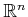. This enables the vector field definition and notation to extend naturally fromto smooth manifolds. A vector field on a manifoldassigns a vector in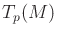for every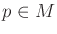. It can once again be imagined as a needle diagram, but now the needle diagram is spread over the manifold, rather than lying in.

The velocity field interpretation of a vector field can also be extended to smooth manifolds. This means that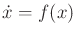now defines a set ofdifferential equations overand is usually expressed using a coordinate neighborhood of the smooth structure. If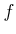is a smooth vector field, then a solution trajectory,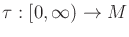, can be defined from any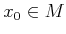. Solution trajectories in the sense of Filipov can also be defined, for the case of piecewise-smooth vector fields.

Steven M LaValle 2020-08-14